## Parsers

 Question 1
Consider the grammar given below:
S → Aa
A → BD
B → b | ε
D → d | ε
Let a, b, d and \$ be indexed as follows:Compute the FOLLOW set of the non-terminal B and write the index values for the symbols in the FOLLOW set in the descending order. (For example, if the FOLLOW set is {a, b, d, \$}, then the answer should be 3210)
 A 30 B 31 C 10 D 21
Compiler-Design       Parsers       GATE 2019       Video-Explanation
Question 1 Explanation:
Follow(B) = First(D) Union Follow(A)
{Follow(B) = Follow(A) when D is epsilon}
Follow(B) = {d} Union {a} = {a,d}
Hence Answer is : 31
 Question 2

Which one of the following kinds of derivation is used by LR parsers?

 A Leftmost in reverse B Rightmost in reverse C Leftmost D Rightmost
Compiler-Design       Parsers       GATE 2019       Video-Explanation
Question 2 Explanation:
LR parsers have Rightmost derivation in reverse.
 Question 3

Consider the augmented grammar given below:

```    S' → S
S → 〈L〉 | id
L → L,S | S
```

Let I0 = CLOSURE ({[S' → ·S]}). The number of items in the set GOTO (I0 , 〈 ) is: _____.

 A 4 B 5 C 6 D 7
Compiler-Design       Parsers       GATE 2019       Video-Explanation
Question 3 Explanation:
I0 = CLOSURE ({[S' → ·S]})Hence, the set GOTO (I0 , 〈 ) has 5 items.
 Question 4
Consider the following grammar:
stmt    →  if expr then expr else expr; stmt | ȯ
expr    →  term relop term | term
term    →  id | number
id      →  a | bc
number  → [0-9]
where relop is a relational operator (e.g., <, >, …), ȯ refers to the empty statement, and if, then, else are terminals.
Consider a program P following the above grammar containing ten if terminals. The number of control flow paths in P is ________. For example, the program
if e1 then e2 else e3 has 2 control flow paths, e1 e2 and e1 e3.

 A 1024 B 1025 C 1026 D 1027
Compiler-Design       Parsers       GATE 2017 [Set-1]       Video-Explanation
Question 4 Explanation:
To get 10 'if' we need to use grammar to get,
if then else ; stmt
if then else ; if then else . stmt
:
:
:
(keep doing 10 times to get 10 'if')
We know that every if statement has 2 control flows as given in question. Hence,
We have 2 control flow choices for 1st 'if'
We have 2 control flow choices for 2nd 'if'
:
:
:
We have 2 control flow choices for 10th 'if'
Since all the choices are in one single structure or combination, so total choices are
2 × 2 × 2 × ........ 10 times = 210 = 1024
 Question 5

Which of the following statements about parser is/are CORRECT?

I. Canonical LR is more powerful than SLR.

II. SLR is more powerful than LALR.

III. SLR is more powerful than Canonical LR.

 A I only B II only C III only D II and III only
Compiler-Design       Parsers       GATE 2017 [Set-2]       Video-Explanation
Question 5 Explanation:
Canonical LR is more powerful than SLR as every grammar which can be parsed by SLR parser, can also be parsed by CLR parser.
The power in increasing order is:
LR(0) < SLR < LALR < CLR
Hence only I is true.
 Question 6

Which one of the following is True at any valid state in shift-reduce parsing?

 A Viable prefixes appear only at the bottom of the stack and not inside B Viable prefixes appear only at the top of the stack and not inside C The stack contains only a set of viable prefixes D The stack never contains viable prefixes
Compiler-Design       Parsers       GATE 2015 [Set-1]
Question 6 Explanation:
A handle is actually on the top of the stack.
A viable prefixes is prefix of the handle and so can never extend to the right of handle, i.e., top of stack.
So set of viable prefixes is in stack.
 Question 7

Among simple LR (SLR), canonical LR, and look-ahead LR (LALR), which of the following pairs identify the method that is very easy to implement and the method that is the most powerful, in that order?

 A SLR, LALR B Canonical LR, LALR C SLR, canonical LR D LALR, canonical LR
Compiler-Design       Parsers       GATE 2015 [Set-3]
Question 7 Explanation:
SLR is very easy to implement and CLR is most powerful method.
 Question 8

Consider the following grammar G.

```  S → F ⎪ H
F → p ⎪ c
H → d ⎪ c```

Where S, F and H are non-terminal symbols, p, d and c are terminal symbols. Which of the following statement(s) is/are correct?

S1: LL(1) can parse all strings that are generated using grammar G.
S2: LR(1) can parse all strings that are generated using grammar
 A Only S1 B Only S2 C Both S1 and S2 D Neither S1 nor S2
Compiler-Design       Parsers       GATE 2015 [Set-3]
Question 8 Explanation:
For LL(1),
For first production,So, there is 'c' common in both the first(s) in the production of S. So not LL(1).
For LR(1),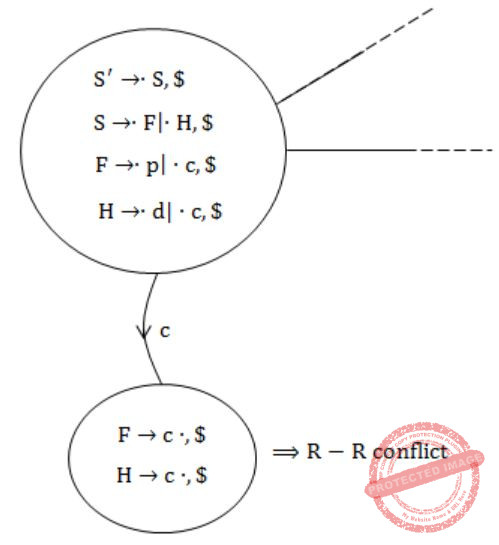Since R-R conflict is present. So, not LR(1).
Hence, Option (D) is the correct answer.
 Question 9

A canonical set of items is given below

```S --> L. > R
Q --> R.```

On input symbol < the set has

 A a shift-reduce conflict and a reduce-reduce conflict. B a shift-reduce conflict but not a reduce-reduce conflict. C a reduce-reduce conflict but not a shift-reduce conflict. D neither a shift-reduce nor a reduce-reduce conflict.
Compiler-Design       Parsers       GATE 2014 [Set-1]
Question 9 Explanation:
The input symbol is “<” which is not in canonical set of item, so it is neither a shift-reduce nor a reduce-reduce conflict with reference to “<” symbol.
But if it would have asked about “>” then it will be a SR conflict.
 Question 10

What is the maximum number of reduce moves that can be taken by a bottom-up parser for a grammar with no epsilon- and unit-production (i.e., of type A → є and A → a) to parse a string with n tokens?

 A n/2 B n-1 C 2n-1 D 2n
Compiler-Design       Parsers       GATE 2013
Question 10 Explanation:
Since it is given that the grammar cannot have:
1) epsilon production
2) production of the form A → a
Consider the grammar:
S → Sa | a
If we were to derive the string “aaa” whose length is 3 then the number of reduce moves that would have been required are shown below:
S → Sa
→ Saa
→ aaa
This shows us that it has three reduce moves. The string length is 3 and the number of reduce moves is also 3. So presence of such kinds of production might give us the answer “n” for maximum number of reduce moves. But these productions are not allowed as per the question.
Also note that if a grammar does not have unit production then the maximum number of reduce moves can not exceed “n” where “n” denotes the length of the string.
3) No unit productions
Consider the grammar:
S → A
A → B
B → C
C → a
If we were to derive the string “a” whose length is 1 then the number of reduce moves that would have been required are shown below:
S → A
A → B
B → C
C → a
This shows us that it has four reduce moves. The string length is 1 and the number of reduce moves is 4. So presence of such kind of productions might give us the answer “n+1” or even more, for maximum number of reduce moves. But these productions are not allowed as per the question.
Now keeping in view the above points suppose we want to parse the string “abcd”. (n = 4) using bottom-up parsing where strings are parsed finding the rightmost derivation of a given string backwards. So here we are concentrating on deriving right most derivations only.
We can write the grammar which accepts this string which in accordance to the question, (i.e., with no epsilon- and unit-production (i.e., of type A → є and A → B) and no production of the form A → a) as follows:
S → aB
B → bC
C → cd
The Right Most Derivation for the above is:
S → aB (Reduction 3)
→ abC (Reduction 2)
→ abcd (Reduction 1)
We can see here the number of reductions present is 3.
We can get less number of reductions with some other grammar which also doesn’t produce unit or epsilon productions or production of the form A → a
S → abA
A → cd
The Right Most Derivation for the above is:
S → abA (Reduction 2)
→ abcd (Reduction 1)
Hence 2 reductions.
But we are interested in knowing the maximum number of reductions which comes from the 1st grammar. Hence total 3 reductions as maximum, which is (n – 1) as n = 4 here.
 Question 11

For the grammar below, a partial LL(1) parsing table is also presented along with the grammar. Entries that need to be filled are indicated as E1, E2, and E3. ε is the empty string, \$ indicates end of input, and, | separates alternate right hand sides of productions.

```S → aAbB | bAaB | ε
A → S
B → S```The FIRST and FOLLOW sets for the non-terminals A and B are

 A FIRST(A) = {a,b,ε} = FIRST(B) FOLLOW(A) = {a,b} FOLLOW(B) = {a,b,\$} B FIRST(A) = {a,b,\$} FIRST(B) = {a,b,ε} FOLLOW(A) = {a,b} FOLLOW(B) = {\$} C FIRST(A) = {a,b,ε} = FIRST(B) FOLLOW(A) = {a,b} FOLLOW(B) = ∅ D FIRST(A) = {a,b} = FIRST(B) FOLLOW(A) = {a,b} FOLLOW(B) = {a,b}
Compiler-Design       Parsers       GATE 2012
Question 11 Explanation:
FIRST (P): is the set of terminals that begin the strings derivable from non terminal P. If P derives epsilon then we include epsilon in FIRST(P).
FOLLOW(P): is the set of terminals that can appear immediately to the right of P in some sentential form.
FIRST(A) = FIRST (S)
FIRST (S) = FIRST (aAbB) and FIRST (bAaB) and FIRST (ϵ)
FIRST(S) = {a, b, ϵ}
FIRST (B) = FIRST (S) = {a, b, ϵ} = FIRST (A)
FOLLOW(A) = {b} // because of production S→a A b B
FOLLOW(A) = {a} // because of production S→ b A a B
So FOLLOW (A) = {a, b}
FOLLOW (B) = FOLLOW (S) // because of production S→ a A b B
FOLLOW (S) = FOLLOW (A) // because of production S → A
So FOLLOW (S) = {\$, a, b} = FOLLOW(B)
 Question 12

For the grammar below, a partial LL(1) parsing table is also presented along with the grammar. Entries that need to be filled are indicated as E1, E2, and E3. ε is the empty string, \$ indicates end of input, and, | separates alternate right hand sides of productions.

```S → aAbB | bAaB | ε
A → S
B → S```The appropriate entries for E1, E2, and E3 are

 A E1: S → aAbB,A → S E2: S → bAaB,B→S E3: B → S B E1: S → aAbB,S→ ε E2: S → bAaB,S → ε E3: S → ε C E1: S → aAbB,S → ε E2: S → bAaB,S→ε E3: B → S D E1: A → S,S →ε E2: B → S,S → ε E3: B →S
Compiler-Design       Parsers       GATE 2012
Question 12 Explanation:
The entries in E1, E2 and E3 is related to S and B, so we have to take only those production which have S and B in LHS.
S→ aAbB | bAaB | ε
The production S→ aAbB will go under column FIRST (aAbB) = a, so S→ aAbB will be in E1.
S→ bAaB will go under column FIRST(bAaB) = b, so S→ bAaB will be in E2.
S→ ε will go under FOLLOW (S) = FOLLOW(B) = {a, b, \$ } , So S→ ε will go in E1, E2 and under column of \$.
So E1 will have: S→ aAbB and S→ ε.
E2 will have S→ bAaB and S→ ε.
Now, B→ S will go under FIRST (S) = {a, b, ε}
Since FIRST(S) = ε so B→ S will go under FOLLOW (B) = {a, b, \$}
So E3 will contain B→ S.
 Question 13

Consider two binary operators ‘↑’ and ‘↓’ with the precedence of operator ↓ being lower than that of the operator ↑. Operator ↑ is right associative while operator ↓, is left associative. Which one of the following represents the parse tree for expression (7↓3↑4↑3↓2)?

 AB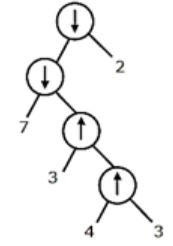C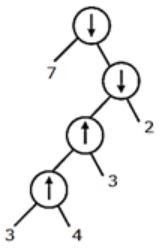DCompiler-Design       Parsers       GATE 2011
Question 13 Explanation:
7 ↓ 3 ↑ 4 ↑ 3 ↓ 2
⇒ 7 ↓ (3 ↑ (4 ↑ 3)) ↓ 2
⇒ 7 ↓ (3 ↑ (4 ↑ 3))) ↓ 2 as ↓ is left associative
 Question 14

The grammar S → aSa|bS|c is

 A LL(1) but not LR(1) B LR(1) but not LR(1) C Both LL(1) and LR(1) D Neither LL(1) nor LR(1)
Compiler-Design       Parsers       GATE 2010
Question 14 Explanation:
The LL(1) parsing table for the given grammar is:As there is no conflict in LL(1) parsing table, hence the given grammar is LL(1) and since every LL(1) is LR(1) also, so the given grammar is LL(1) as well as LR(1).
 Question 15

Which of the following statements are TRUE?

I.There exist parsing algorithms for some programming languages whose complexities are less than θ(n3).
II.A programming language which allows recursion can be implemented with static storage.
III.No L-attributed definition can be evaluated in the framework of bottom-up parsing.
IV.Code improving transformations can be performed at both source language and intermediate code level.
 A I and II B I and IV C III and IV D I, III and IV
Compiler-Design       Parsers       GATE 2009
Question 15 Explanation:
Statement II is false, as a programming language which allows recursion requires dynamic storage allocation. Statement III is false, as L-attributed definition (assume for instance the L-attributed definition has synthesized attribute only) can be evaluated in bottom up framework.
Statement I is true, as the bottom up and top down parser take O(n) time to parse the string , i.e. only one scan of input is required.
Statement IV is true,Code improving transformations can be performed at both source language and intermediate code level. For example implicit type casting is also a kind of code improvement which is done during semantic analysis phase and intermediate code optimization is a topic itself which uses various techniques to improve the code such as loop unrolling, loop invariant.
 Question 16

Which of the following describes a handle (as applicable to LR-parsing) appropriately?

 A It is the position in a sentential form where the next shift or reduce operation will occur. B It is non-terminal whose production will be used for reduction in the next step. C It is a production that may be used for reduction in a future step along with a position in the sentential form where the next shift or reduce operation will occur. D It is the production p that will be used for reduction in the next step along with a position in the sentential form where the right hand side of the production may be found.
Compiler-Design       Parsers       GATE 2008
Question 16 Explanation:
A handle is the production p that will be used for reduction in the next step along with a position in the sentential form where the right hand side of the production may be found.
 Question 17

Which one of the following is a top-down parser?

 A Recursive descent parser. B Operator precedence parser. C An LR(k) parser. D An LALR(k) parser.
Compiler-Design       Parsers       GATE 2007
Question 17 Explanation:
Recursive descent parser is top down parser, while others are bottom up parser.
 Question 18

Consider the grammar with non-terminals N = {S,C,S1},terminals T = {a,b,i,t,e}, with S as the start symbol, and the following set of rules:

```      S --> iCtSS1|a
S1 --> eS|ϵ
C --> b```

The grammar is NOT LL(1) because:

 A it is left recursive B it is right recursive C it is ambiguous D it is not context-free
Compiler-Design       Parsers       GATE 2007
Question 18 Explanation:
The given grammar is not left recursive and also it is context free (Type 2 grammar), so option A and D is wrong. Being a right recursive grammar is not an issue for LL(1) grammar. So even if given grammar is right recursive, this is not a reason for NOT LL(1).
This grammar has two parse tree for string “ibt ibt aea”.Question 19

Consider the following two statements:

```P: Every regular grammar is LL(1)
Q: Every regular set has a LR(1) grammar```

Which of the following is TRUE?

 A Both P and Q are true B P is true and Q is false C P is false and Q is true D Both P and Q are false
Compiler-Design       Parsers       GATE 2007
Question 19 Explanation:
Every regular grammar is LL(1) is false, as the grammar may have left recursion or left factoring or also it is possible that grammar is ambiguous.
For ex: Consider a regular grammar
S -> aS | a | ϵ
this grammar is ambiguous as for string "a" two parse tree is possible.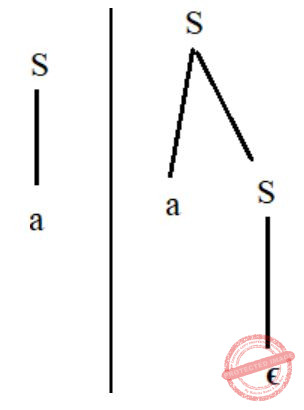Hence it is regular but not LL(1).
But every regular set has a language accept or as DFA , so every regular set must have atleast one grammar which is unambiguous.
Hence, every regular set has LR(1) grammar.
 Question 20

Consider the following grammar.

```   S → S * E
S → E
E → F + E
E → F
F → id ```

Consider the following LR(0) items corresponding to the grammar above.

```(i) S → S * .E
(ii) E → F. + E
(iii) E → F + .E ```

Given the items above, which two of them will appear in the same set in the canonical sets-of-items for the grammar?

 A (i) and (ii) B (ii) and (iii) C (i) and (iii) D None of the above
Compiler-Design       Parsers       GATE 2006
Question 20 Explanation:
As we can see in the below given LR(0) items, that all three belongs to different state (sets).Question 21

Consider the following grammar:

```   S → FR
R → S | ε
F → id```

In the predictive parser table, M, of the grammar the entries M[S,id] and M[R,\$] respectively.

 A {S → FR} and {R → ε} B {S → FR} and { } C {S → FR} and {R → *S} D {F → id} and {R → ε}
Compiler-Design       Parsers       GATE 2006
Question 21 Explanation:
Predictive parsing table for the mentioned grammar: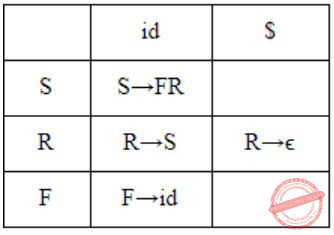The representation M[X,Y] means X represents Variable (rows) and Y represents terminals (columns).
The productions are filled in parsing table by the below mentioned rules:
For every production P → α, we have:
Rule 1: If P → α is a production then add this production for each terminal “t” which is in FIRST of [α] i.e., ADD P → α to M[P, a]
Rule 2: If “ϵ” belongs to FIRST of [P] then add P → α to M[P, b] where “b” represents terminals FOLLOW[P].
By the above rules, we can see that production S → FR will go M[S, a] where “a” is FIRST [FR] which is equal to FIRST[F] = id, So S → FR will go in M[S,id].
Since in the production R→ϵ , FIRST[ϵ] = ϵ, hence the production will go in M[R, b] where “b” represents terminals FOLLOW[R] and FOLLOW[R] = \$, so production R→ϵ will go in M[R,\$]
 Question 22

The grammar A → AA | (A) | ε is not suitable for predictive-parsing because the grammar is:

 A ambiguous B left-recursive C right-recursive D an operator-grammar
Compiler-Design       Parsers       GATE 2005
Question 22 Explanation:
The given grammar can be turned into a infinite parse tree. So it is ambiguous.
It have A → AA has left recursion.
 Question 23

Consider the grammar:

`   S → (S) | a `

Let the number of states in SLR(1), LR(1) and LALR(1) parsers for the grammar be n1, n2 and n3 respectively. The following relationship holds good:

 A n1 < n2 < n3 B n1 = n3 < n2 C n1 = n2 = n3 D n1 ≥ n3 ≥ n2
Compiler-Design       Parsers       GATE 2005
Question 23 Explanation:
→ SLR(1) and LALR(1) both are be the states of LR(0) items then SLR(1) = LALR(1).
→ LR(1) be the states of LR(1) items.
→ LR(0) items never be greater than LR(1) items then SLR(1) = LALR(1) < LR(1)
n1 = (n3) < (n2)
 Question 24

Consider the following expression grammar. The seman­tic rules for expression calculation are stated next to each grammar production.

```E → number 	 E.val = number. val
|E '+' E 	 E(1).val = E(2).val + E>sup>(3).val
|E '×' E	 E(1).val = E(2).val × E(3).val```

Assume the conflicts in Part (a) of this question are resolved and an LALR(1) parser is generated for parsing arithmetic expressions as per the given grammar. Consider an expression 3 × 2 + 1. What precedence and associativity properties does the generated parser realize?

 A Equal precedence and left associativity; expression is evaluated to 7 B Equal precedence and right associativity; expression is evaluated to 9 C Precedence of '×' is higher than that of '+', and both operators are left associative; expression is evaluated to 7 D Precedence of '+' is higher than that of '×', and both operators are left associative; expression is evaluated to 9
Compiler-Design       Parsers       GATE 2005
Question 24 Explanation:
First of all, it is ambiguous grammar. Hence, equal precedence and associativity. Now as Yacc resolved it with shift move we will shift until the last operator and then we will start reducing.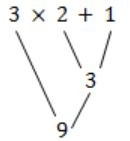Hence, the answer is 9 and right associative.
 Question 25

Which of the following grammar rules violate the requirements of an operator grammar? P,Q,R are nonterminals, and r,s,t are terminals.

(i) P → Q R
(ii) P → Q s R
(iii) P → ε
(iv) P → Q t R r
 A (i) only B (i) and (iii) only C (ii) and (iii) only D (iii) and (iv) only
Compiler-Design       Parsers       GATE 2004
Question 25 Explanation:
Operator values doesn't contains nullable values and two adjacent non-terminals on RHS production.
i) On RHS it contains two adjacent non-terminals.
ii) Have nullable values.
 Question 26

Assume that the SLR parser for a grammar G has n1 states and the LALR parser for G has n2 states. The relationship between n1 and n2 is:

 A n1 is necessarily less than n2 B n1 is necessarily equal to n2 C n1 is necessarily greater than n2 D None of the above
Compiler-Design       Parsers       GATE 2003
Question 26 Explanation:
No. of states in SLR and LALR are equal and no. of states in SLR and LALR are less than or equal to LR(1).
 Question 27

Consider the grammar shown below

```S → i E t S S' | a
S' → e S | ε
E → b ```

In the predictive parse table. M, of this grammar, the entries M[S', e] and M[S', \$] respectively are

 A {S'→e S} and {S'→ε} B {S'→e S} and { } C {S'→ε} and {S'→ε} D {S'→e S, S'→ε} and {S'→ε}
Compiler-Design       Parsers       GATE 2003
Question 27 Explanation:
First(S) = {1,a}
First(S') = {e,ε}
First(E) = {b}
Follow(S') = {e,\$}
Only when 'First' contains ε, we need to consider FOLLOW for getting the parse table entry.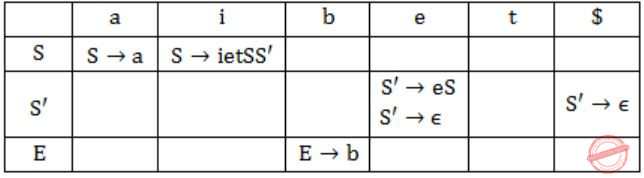Hence, option (D) is correct.
 Question 28

Consider the grammar shown below.

```S → C C
C → c C | d```

The grammar is

 A LL(1) B SLR(1) but not LL(1) C LALR(1) but not SLR(1) D LR(1) but not LALR(1)
Compiler-Design       Parsers       GATE 2003
Question 28 Explanation: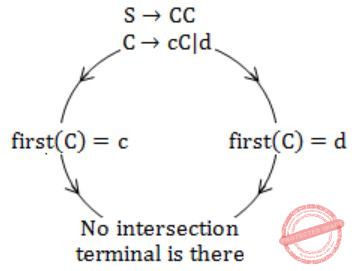Hence, it is LL(1).
 Question 29

Which of the following statements is false?

 A An unambiguous grammar has same leftmost and rightmost derivation B An LL(1) parser is a top-down parser C LALR is more powerful than SLR D An ambiguous grammar can never be LR(k) for any k
Compiler-Design       Parsers       GATE 2001
Question 29 Explanation:
Option B: LL parser is a top-down parser for a subset of context-free languages. It parses the input from Left to right, performing Left most derivation of the sentence.
Option C: LALR is more powerful than SLR.
Option D: An ambiguous grammar can never be LR (k) for any k, because LR(k) algorithm aren’t designed to handle ambiguous grammars. It would get stuck into undecidability problem, if employed upon an ambiguous grammar, no matter how large the constant k is.
 Question 30

Consider the following grammar with terminal alphabet ∑{a,(,),+,*} and start symbol E. The production rules of the grammar are:

```              E → aA
E → (E)
A → +E
A → *E
A → ε ```

(a) Compute the FIRST and FOLLOW sets for E and A.
(b) Complete the LL(1) parse table for the grammar.

 A Theory Explanation is given below.
Compiler-Design       Parsers       GATE 2001
 Question 31

Which of the following derivations does a top-down parser use while parsing an input string? The input is assumed to be scanned in left to right order.

 A Leftmost derivation B Leftmost derivation traced out in reverse C Rightmost derivation D Rightmost derivation traced out in reverse
Compiler-Design       Parsers       GATE 2000
Question 31 Explanation:
Top-down parser - Leftmost derivation
Bottom-Up parser - Reverse of rightmost derivation
 Question 32

Which of the following is the most powerful parsing method?

 A LL (1) B Canonical LR C SLR D LALR
Compiler-Design       Parsers       GATE 1999
Question 32 Explanation:
Canonical LR is most powerful.
LR > LALR > SLR
 Question 33

Which of the following statements is true?

 A SLR parser is more powerful than LALR B LALR parser is more powerful than Canonical LR parser C Canonical LR parser is more powerful than LALR parser D The parsers SLR, Canonical CR, and LALR have the same power
Compiler-Design       Parsers       GATE 1998
Question 33 Explanation:
LR > LALR > SLR
Canonical LR parser is more powerful than LALR parser.
 Question 34

A shift reduce parser carries out the actions specified within braces immediately after reducing with the corresponding rule of grammar

```S → xxW {print "1"}
S → y {print "2"}
W → Sz {print "3"} ```

What is the translation of xxxxyzz using the syntax directed translation scheme described by the above rules?

 A 23131 B 11233 C 11231 D 33211
Compiler-Design       Parsers       GATE 1995
Question 34 Explanation:⇒ 23131
Note SR is bottom up parser.
 Question 35

Consider the following grammar.

```     S → aSB|d
B → b ```

The number of reduction steps taken by a bottom-up parser while accepting the string aaadbbb is _______.

 A 7
Compiler-Design       Parsers       GATE 2020
Question 35 Explanation:7 reductions total.
 Question 36

Consider the SLR(1) and LALR (1) parsing tables for a context free grammar. Which of the following statements is/are true?

 A The go to part of both tables may be different. B The shift entries are identical in both the tables. C The reduce entries in the tables may be different. D The error entries in the tables may be different. E B, C and D.
Compiler-Design       Parsers       GATE 1992
Question 36 Explanation:
Goto parts and shift entry must be same.
Reduce entry and error entry may be different due to conflicts.
 Question 37

Choose the correct alternatives (more than one may be correct) and write the corresponding letters only: Indicate all the true statements from the following:

 A Recursive descent parsing cannot be used for grammar with left recursion. B The intermediate form the representing expressions which is best suited for code optimization is the post fix form. C A programming language not supporting either recursion or pointer type does not need the support of dynamic memory allocation. D Although C does not support call by name parameter passing, the effect can be correctly simulated in C. E No feature of Pascal violates strong typing in Pascal. F A and D
Compiler-Design       Parsers       GATE 1991
Question 37 Explanation:
(A) It is true. Left recursive grammar if used directly in recursive descent parsing causes an infinite loop. So, left recursion must be removed before giving to a recursive descent parser.
(B) False.
(C) It is false. The language can have dynamic data types which required dynamically growing memory when data type size increases.
(D) Is true and using macro we can do this.
(E) Out of syllabus now.
 Question 38

Merging states with a common core may produce __________ conflicts and does not produce ___________ conflicts in an LALR purser.

 A Reduce-Reduce, Shift-Reduce
Compiler-Design       Parsers       GATE 1989
Question 38 Explanation:
Merge states with a common core may produce Reduce-Reduce conflicts and does not produce Shift-Reduce conflicts in an LALR parser.
 Question 39

An operator precedence parser is a

 A Bottom-up parser. B Top-down parser. C Back tracking parser. D None of the above.
Compiler-Design       Parsers       GATE 1987
Question 39 Explanation:
An operator precedence parser is a Bottom-up parser.
 Question 40
Consider the following statements.
S1:Every SLR(1) grammar is unambiguous but there are certain unambiguous grammars that  are not SLR(1).
S2: For any context-free grammar, there is a parser that takes at most O(n3 )
Which one of the following options is correct?
 A S1is true and S2is true B S1is true and S2is false C S1is false and S2is true D S1is false and S2is false
Compiler-Design       Parsers       GATE 2021 CS-Set-1
Question 40 Explanation:

Every unambiguous grammar need not be SLR(1). As we know some unambiguous grammar which is CLR(1)  but not SLR(1).  So S1 is true.

Any CFG (which is in CNF form) can be parsed by CYK algorithm in O(n3) where n is length of string. Although it is not given that CFG is in CNF form but since we can convert any CFG in CNF form so S2 is true

 Question 41
Consider the following context-free grammar where the set of terminals is {a, b, c, d, f}Which one of the following choices represents the correct combination for the numbered cells in the parsing table (“blank” denotes that the corresponding cell is empty)?
 A ① S ⟶ Rf ② S ⟶ Rf ③ T ⟶ ∊ ④ T ⟶ ∊ B ① blank ② S ⟶ Rf ③ T ⟶ ∊ ④ T ⟶ ∊ C ① S ⟶ Rf ② blank ③ blank ④ T ⟶ ∊ D ① blank ② S ⟶ Rf ③ blank ④ blank
Compiler-Design       Parsers       GATE 2021 CS-Set-1
Question 41 Explanation:Question 42
Consider the following augmented grammar with {#, @, <, >, a, B, c} as the set of terminals.
S’ ⟶ S
S ⟶ S#cS
S ⟶ SS
S ⟶ S@
S ⟶ < S >
S ⟶ a
S ⟶ b
S ⟶ c
Let I0=CLOSURE({S' ⟶.S}). The number of items in the set GOTO(GOTO(I0, <), <) is ____________.
 A 8
Compiler-Design       Parsers       GATE 2021 CS-Set-2
Question 42 Explanation:Question 43
Which one of the following statements is TRUE?
 A The LALR (1) parser for a grammar G cannot have reduce-reduce conflict if the LR (1) parser for G does not have reduce-reduce conflict. B Symbol table is accessed only during the lexical analysis phase. C Data flow analysis is necessary for run-time memory management. D LR (1) parsing is sufficient for deterministic context-free languages.
Compiler-Design       Parsers       GATE 2022       Video-Explanation
Question 43 Explanation:
Even though there is no reduce-reduce conflict in CLR(1) but in LALR(1) while merging the states differ in only lookahead may get reduce-reduce conflict. So the given statement is not true.
Symbol table is accessed in all the phases of compiler and not only in lexical analysis phase.
Data flow analysis is done in the control flow graph, in the code optimization phase. If LR(1) parses a grammar then definitely it is DCFL, so LR(1) parsing is sufficient for deterministic context-free languages.
 Question 44
Consider the augmented grammar with { + , *, (, ), id } as the set of terminals.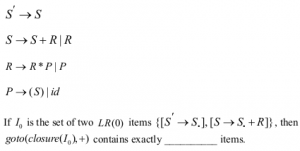A 5
Compiler-Design       Parsers       GATE 2022
Question 44 Explanation:Question 45
Consider the following grammar along with translation rules.A 80
Compiler-Design       Parsers       GATE 2022
Question 45 Explanation:Question 46
Recursive descent parsing is an example of
 A Top-down parsers B Bottom-up parsers C Predictive parsers D None of the above
Compiler-Design       Parsers       ISRO-2016
Question 46 Explanation:
→ A recursive descent parser is a kind of top-down parser built from a set of mutually recursive procedures (or a non-recursive equivalent) where each such procedure implements one of the nonterminals of the grammar.
→ Thus the structure of the resulting program closely mirrors that of the grammar it recognizes.
 Question 47
A top-down parser generates
 A Rightmost Derivation B Rightmost derivation in reverse C Leftmost derivation D Leftmost derivation in reverse
Compiler-Design       Parsers       ISRO-2016
Question 47 Explanation:
→ Top-down parsing can be viewed as an attempt to find leftmost derivations of an input-stream by searching for parse-trees using a top-down expansion of the given formal grammar rules.
→ The inclusive choice is used to accommodate ambiguity by expanding all alternative right-hand-sides of grammar rules.
 Question 48
Shift reduce parsing belongs to a class of
 A bottom up parsing B top down parsing C recursive parsing D predictive parsing
Compiler-Design       Parsers       ISRO CS 2013
Question 48 Explanation:
→ A shift-reduce parser is a class of efficient, table-driven bottom-up parsing methods for computer languages and other notations formally defined by a grammar.
→ The parsing methods most commonly used for parsing programming languages, LR parsing and its variations, are shift-reduce methods.Question 49
Which of the following productions eliminate left recursion in the productions given below:
S → Aa | b
A → Ac | Sd | ε
 A S → Aa | b A → bdA’ A’ → A’c | A’ba | A | ε B S → Aa | b A → A’ | bdA’, A’ → cA’ | adA’ | ε C S → Aa | b A → A’c | A’d A’ → bdA’ | cA | ε D S → Aa | b A → cA’ | adA’ | bdA’ A’ → A | ε
Compiler-Design       Parsers       ISRO CS 2013
Question 49 Explanation:Question 50
YACC builds up
 A SLR parsing table B Canonical LR parsing table C LALR parsing table D None of these
Compiler-Design       Parsers       Nielit Scientist-C 2016 march
Question 50 Explanation:
● YACC (Yet Another Compiler-Compiler) is a computer program.
● It is a Look Ahead Left-to-Right (LALR) parser generator, generating a parser, the part of a compiler that tries to make syntactic sense of the source code, specifically a LALR parser, based on an analytic grammar written in a notation similar to Backus–Naur Form (BNF)
 Question 51
What is the maximum number of reduce moves that can be taken by a bottom up parser for a grammar with no epsilon and unit production(i.e., of type A →   and A →  a) to parse a string with n tokens?
 A n/2 B n-1 C 2n-1 D 2n
Compiler-Design       Parsers       Nielit Scientist-B CS 22-07-2017
Question 51 Explanation:
Since it is given that the grammar cannot have:
1) epsilon production
2) production of the form A → a
Consider the grammar:
S → Sa | a
If we were to derive the string “aaa” whose length is 3 then the number of reduce moves that would have been required are shown below:
S→ Sa
→Saa
→aaa
This shows us that it has three reduce moves. The string length is 3 and the number of reduce moves is also 3. So presence of such kinds of production might give us the answer “n” for maximum number of reduce moves. But these productions are not allowed as per the question.
Also note that if a grammar does not have unit production then the maximum number of reduce moves can not exceed “n” where “n” denotes the length of the string.
3) No unit productions
Consider the grammar:
S→ A
A→ B
B→C
C→a
If we were to derive the string “a” whose length is 1 then the number of reduce moves that would have been required are shown below:
S→ A
A→ B
B→C
C→a
This shows us that it has four reduce moves. The string length is 1 and the number of reduce moves is 4. So presence of such kind of productions might give us the answer “n+1” or even more, for maximum number of reduce moves. But these productions are not allowed as per the question.
Now keeping in view the above points suppose we want to parse the string “abcd”. (n = 4) using bottom-up parsing where strings are parsed finding the rightmost derivation of a given string backwards. So here we are concentrating on deriving rightmost derivations only.
We can write the grammar which accepts this string which in accordance to the question, (i.e., with no epsilon- and unit-production (i.e., of type A → є and A → B) and no production of the form A→a) as follows:
S→aB
B→bC
C→cd
The Right Most Derivation for the above is:
S → aB (Reduction 3)
→ abC (Reduction 2)
→ abcd (Reduction 1)
We can see here the number of reductions present is 3.
We can get less number of reductions with some other grammar which also doesn’t produce unit or epsilon productions or production of the form A→a:
S→abA
A→ cd
The Right Most Derivation for the above is:
S → abA (Reduction 2)
→ abcd (Reduction 1)
Hence 2 reductions.
But we are interested in knowing the maximum number of reductions which comes from the 1st grammar. Hence total 3 reductions as maximum, which is (n – 1) as n = 4 here.
 Question 52

The grammar S ⟶ (S) | SS | ∈ is not suitable for predictive parsing because the grammar is

 A An Operator Grammar B Right Recursive C Left Recursive D Ambiguous
Compiler-Design       Parsers       UGC-NET CS 2018 DEC Paper-2
Question 52 Explanation:
The grammar is ambiguous, as to derive string ( )( )( ) more than one parse tree exists.Question 53

Consider the following Grammar G :

```S➝ A | B
A➝ a | c
B➝ b | c```

Where {S,A,B} is the set of non-terminals, {a,b,c} is the set of terminals.

Which of the following statement(s) is/are correct ?

S1 : LR(1) can parse all strings that are generated using grammar G.
S2 : LL(1)  can parse all strings that are generated using grammar G.

Choose the correct answer from the code given below :

Code :
 A Both S1 and S2 B Only S2 C Neither S1 nor S2 D Only S1
Compiler-Design       Parsers       UGC-NET CS 2018 DEC Paper-2
Question 53 Explanation:
For generating string “c” we have two different parse trees.Since the grammar is Ambiguous so the strings generated by the grammar G can’t be parsed by LR(1) or LL(1) parser.
 Question 54
Which grammar rules violate the requirement of the operator grammar? A, B, C are variables and a, b, c are terminals 1) A → BC 2) A → CcBb 3) A → BaC 4) A → ε
 A 1 only B 1 and 2 only C 1 and 3 only D 1 and 4 only
Compiler-Design       Parsers       ISRO CS 2015
Question 54 Explanation:
Operator values doesn't contains nullable values and two adjacent non-terminals on RHS production.
i) On RHS it contains two adjacent non-terminals.
ii) Have nullable values.
 Question 55
Which one of the following is a top-down parser?
 A Recursive descent parser B Shift left associative parser C SLR(k) parser D LR(k) parser
Compiler-Design       Parsers       ISRO CS 2015
Question 55 Explanation: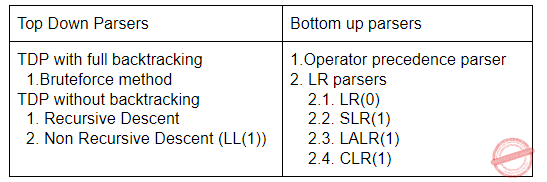Question 56
YACC stands for
 A yet accept compiler constructs B yet accept compiler compiler C yet another compiler construct D yet another compiler compiler
Compiler-Design       Parsers       ISRO CS 2015
Question 56 Explanation:
→ Yacc (Yet Another Compiler-Compiler) is a computer program for the Unix operating system developed by Stephen C. Johnson.
→ It is a Look Ahead Left-to-Right (LALR) parser generator, generating a parser, the part of a compiler that tries to make syntactic sense of the source code, specifically a LALR parser, based on an analytic grammar written in a notation similar to Backus-Naur Form (BNF)
 Question 57
Which statement is true?
 A LALR parser is more powerful and costly as compare to other parsers B All CFG’s are LP and not all grammars are uniquely defined C Every SLR grammar is unambiguous but not every unambiguous grammar is SLR D LR(K) is the most general backtracking shift reduce parsing method
Compiler-Design       Parsers       ISRO CS 2015
Question 57 Explanation:

Option-A: LR > LALR > SLR

Canonical LR parser is more powerful than LALR parser. So, it FALSE

Option-B: Here, LP is linear precedence. Every grammar generated by LP are CFG but all CFG's are not LP. So, it is false

Option-C: TRUE

Option-D: LR(K) is general non backtracking shift reduce parsing method. So, It is false
 Question 58

With respect to compiler design, "recursive descent" is a ____ parsing technique that reads the inputs from ____.

 A top-down, right to left B top-down, left to right C bottom up, right to left D bottom up, left to right
Compiler-Design       Parsers       JT(IT) 2018 PART-B Computer Science
Question 58 Explanation:
A recursive descent parser is a kind of top-down parser built from a set of mutually recursive procedures (or a non-recursive equivalent) where each such procedure implements one of the nonterminals of the grammar. Thus the structure of the resulting program closely mirrors that of the grammar it recognizes.
→ Top down parsers reads the input from left to right and bottom up parsers are reads the input from left to right and reverse.
 Question 59

Which of the following is NOT a bottom up, shift reduce parser?

 A LR parser B LL parser C SLR parser D LALR parser
Compiler-Design       Parsers       JT(IT) 2018 PART-B Computer Science
Question 59 Explanation:
Bottom Up SR Parsers are:
1. SLR
2. LALR
3. CLR
4. LR(0)
Top Down parser:
1. Recursive descent
2. Non Recursive descent(LL(1))
 Question 60

Which of the following phases of the compilation process is also known as parsing?

 A Lexical analysis B Code optimization C Syntax analysis D Semantic analysis
Compiler-Design       Parsers       JT(IT) 2018 PART-B Computer Science
Question 60 Explanation:
Parsing, syntax analysis, or syntactic analysis is the process of analysing a string of symbols, either in natural language, computer languages or data structures, conforming to the rules of a formal grammar. The term parsing comes from Latin pars (orationis), meaning part (of speech).
 Question 61
Consider an ε-tree CFG. If for every pair of productions A → u and A → v
 A if FIRST(u) ∩ FIRST(v) is empty then the CFG has to be LL(1) B If the CFG is LL(1) then FIRST(u) ∩ FIRST(v) has to be empty C Both (A) and (B) D None of the above
Compiler-Design       Parsers       Nielit Scientific Assistance CS 15-10-2017
Question 61 Explanation:
The condition for a grammar to be LL(1)
Theorem: A context free grammar G=(V​ T​ , V​ N​ , S,P) is LL(1) if and if only if for every nonterminal A and every strings of symbols ⍺,β such that ⍺≠β and A → ⍺,β we have
1. First(⍺) ∩ First (β) ∩ Follow(A)=Θ.
2. If ⍺ *⇒ ε then First(β) ∩ Follow(A)= Θ.
If grammar is epsilon free then condition 2 is not required.
Now as per condition 1, for every non-terminal A, if we have A → u and A→v
And First(u) First(v) = φ and CFG is epsilon free then it must be LL(1) and if epsilon free CFG is LL(1) then it must satisfy the condition 1.
 Question 62
Which of the following is FALSE?
 A The grammar S → a Sb |bSa|SS|∈, where S is the only non-terminal symbol and ∈ is the null string, is ambiguous. B SLR is powerful than LALR. C An LL(1) parser is a top-down parser. D YACC tool is an LALR(1) parser generator.
Compiler-Design       Parsers       UGC NET CS 2016 July- paper-2
Question 62 Explanation:
A grammar is said to be ambiguous if and only if it can generate parse trees of both ​ Left ​ Most Derivation or Right Most Derivation.
In this grammar we are having two different parse trees For string "aabb" using leftmost derivation only.Statement B is wrong because in LALR we use lookahead symbols to put the reduce entries into the parsing table. Because of which number of blank entries in LALR parser are more than that of SLR parser which in turn increases the error detection capability of LALR parser. So LALR parser is more powerful than SLR.
Statement C is true because LL(1) parser is a top-down parser
Statement D is also true because YACC(Yet Another Compiler Compiler) is a tool which generates LALR parser for a given grammar.
 Question 63
Which of the following statements is false?
 A Top-down parsers are LL parsers where first L stands for left - to - right scan and second L stands for a leftmost derivation. B (000)* is a regular expression that matches only strings containing an odd number of zeroes, including the empty string. C Bottom-up parsers are in the LR family, where L stands for left - to - right scan and R stands for rightmost derivation. D The class of context - free languages is closed under reversal. That is, if L is any context- free language, then the language L​ R​ = {w​ R​ : w∈L} is context - free.
Computer-Organization       Parsers       UGC NET CS 2015 Dec- paper-2
Question 63 Explanation:
TRUE: Top-down parsers are LL parsers where first L stands for left - to - right scan and second L stands for a leftmost derivation.
FALSE: (000)* is a regular expression that matches any string containing at least 3 number of zeroes, including the empty string.
TRUE: Bottom-up parsers are in the LR family, where L stands for left - to - right scan and R stands for rightmost derivation.
TRUE: The class of context - free languages is closed under reversal. That is, if L is any context - free language, then the language L​ R​ = {w​ R​ : w∈L} is context - free.
 Question 64
Which one from the following is false?
 A LALR parser is Bottom up parser B A parsing algorithm which performs a left to right scanning and a right most deviation is RL (1) C LR parser is Bottom up parser. D In LL(1), the 1 indicates that there is a one - symbol look - ahead.
Compiler-Design       Parsers       UGC NET CS 2015 Jun- paper-2
Question 64 Explanation:
TRUE: LALR parser is Bottom up parsers.
TRUE: LR parser is Bottom up parser. It has SLR, LALR and SLR.
TRUE: In LL(1), the 1 indicates that there is a one - symbol look - ahead.
FALSE: A parsing algorithms scans right to left and reverse order.
 Question 65
A Top-down Parser generates :
 A Leftmost derivation B Rightmost derivation C Rightmost derivation in reverse D Leftmost derivation in reverse
Compiler-Design       Parsers       UGC NET CS 2005 Dec-Paper-2
Question 65 Explanation:
→ Top-down parsing can be viewed as an attempt to find leftmost derivations of an input-stream by searching for parse-trees using a top-down expansion of the given formal grammar rules.
→ Inclusive choice is used to accommodate ambiguity by expanding all alternative right-hand-sides of grammar rules.
 Question 66
The ‘K’ in LR(R) cannot be :
 A 0 B 1 C 2 D None of these
Compiler-Design       Parsers       UGC NET CS 2006 Dec-paper-2
Question 66 Explanation:
→ The name LR is often followed by a numeric qualifier, as in LR(1) or sometimes LR(k). To avoid backtracking or guessing, the LR parser is allowed to peek ahead at k lookahead input symbols before deciding how to parse earlier symbols.
→ Typically k is 1 and is not mentioned. The name LR is often preceded by other qualifiers, as in SLR and LALR. The LR(k) condition for a grammar was suggested by Knuth to stand for "translatable from left to right with bound k."
 Question 67
YACC builds up __________ parsing table.
 A LALR B LR C SLR D LLR
Compiler-Design       Parsers       UGC NET CS 2006 June-Paper-2
Question 67 Explanation:
Yacc (Yet Another Compiler-Compiler):
It is a Look Ahead Left-to-Right (LALR) parser generator, generating a parser, the part of a compiler that tries to make syntactic sense of the source code, specifically a LALR parser, based on an analytic grammar written in a notation similar to Backus–Naur Form (BNF).
**YACC builds up LALR parsing table.
 Question 68
The action of passing the source program into the proper syntactic class is known as :
 A Syntax analysis B Lexical analysis C Interpretation analysis D Uniform symbol generation
Compiler-Design       Parsers       UGC NET CS 2006 June-Paper-2
Question 68 Explanation:
Lexical analysis is the first phase of a compiler. It takes the modified source code from language preprocessors that are written in the form of sentences. The lexical analyzer breaks these syntaxes into a series of tokens, by removing any whitespace or comments in the source code.
 Question 69
Shift-Reduce parsers perform the following :
 A Shift step that advances in the input stream by K(K > 1) symbols and Reduce step that applies a completed grammar rule to some recent parse trees, joining them together as one tree with a new root symbol. B Shift step that advances in the input stream by one symbol and Reduce step that applies a completed grammar rule to some recent parse trees, joining them together as one tree with a new root symbol. C Shift step that advances in the input stream by K(K = 2) symbols and Reduce step that applies a completed grammar rule to form a single tree. D Shift step that does not advance in the input stream and Reduce step that applies a completed grammar rule to form a single tree.
Compiler-Design       Parsers       UGC NET CS 2014 Dec-Paper-2
Question 69 Explanation:
Shift-Reduce parsers perform shift step that advances in the input stream by one symbol and Reduce step that applies a completed grammar rule to some recent parse trees, joining them together as one tree with a new root symbol.
 Question 70
Which of the following is true ?
 A Canonical LR parser is LR (1) parser with single look ahead terminal B All LR(K) parsers with K > 1 can be transformed into LR(1) parsers. C Both (A) and (B) D None of the above
Compiler-Design       Parsers       UGC NET CS 2014 Dec-Paper-2
Question 70 Explanation:
TRUE: Canonical LR parser is LR (1) parser with single look ahead terminal
TRUE: All LR(K) parsers with K > 1 can be transformed into LR(1) parsers.
 Question 71
A grammar G is LL(1) if and only if the following conditions hold for two distinct productions A → α | β
1. First (α) ∩ First (β) ≠ {a} where a is some terminal symbol of the grammar.
2. First (α) ∩ First (β) ≠ λ
III. First (α) ∩ Follow (A) = φ if λ ∈ First (β)
 A I and II B I and III C II and III D I, II and III
Compiler-Design       Parsers       UGC NET CS 2014 June-paper-2
Question 71 Explanation:
A grammar G is LL(1) if and only if the following conditions hold for two distinct productions:
A → α | β
1. First (α) and First (β) must be disjoint if none of α and β contains NULL move.
2. At most one of the strings α or β can drive NULL move i.e. α → NULL(since First (α) and First (β) are disjoint). In this case, First (β) and Follow(A) must be disjoint.
Hence the answer is option(D).
 Question 72
Which of the following suffices to convert an arbitrary CFG to an LL(1) grammar ?
 A Removing left recursion alone B Removing the grammar alone C Removing left recursion and factoring the grammar D None of the above
Compiler-Design       Parsers       UGC NET CS 2014 June-paper-2
Question 72 Explanation:
→ Left recursion removing (or) factoring the given grammar are not sufficient to convert an arbitrary CFG to an LL(1) grammar.
→ To convert an arbitrary CFG to an LL(1) grammar we need to remove the left recursion and as well as left factoring without that we cannot convert.
 Question 73
A shift reduce parser suffers from
 A shift reduce conflict only B reduce reduce conflict only C both shift reduce conflict and reduce reduce conflict D shift handle and reduce handle conflicts
Compiler-Design       Parsers       UGC NET CS 2014 June-paper-2
Question 73 Explanation:
→ A shift-reduce parser scans and parses the input text in one forward pass over the text, without backing up. (That forward direction is generally left-to-right within a line, and top-to-bottom for multi-line inputs.) The parser builds up the parse tree incrementally, bottom up, and left to right, without guessing or backtracking.
A shift-reduce parser works by doing some combination of Shift steps and Reduce steps, hence the name.
→ A Shift step advances in the input stream by one symbol. That shifted symbol becomes a new single-node parse tree.
→ A Reduce step applies a completed grammar rule to some of the recent parse trees, joining them together as one tree with a new root symbol.
*** A shift reduce parser suffers from both shift reduce conflict and reduce reduce conflict.
 Question 74
The grammar S ⟶ (S) | SS | ∈ is ​ not​ suitable for predictive parsing because the grammar is
 A An Operator Grammar B Right Recursive C Left Recursive D Ambiguous
Compiler-Design       Parsers       UGC NET CS 2018-DEC Paper-2
Question 74 Explanation:
The grammar is ambiguous, as to derive string ()()() more than one parse tree exists.Question 75
Consider the following Grammar G :
S ➝ A | B
A➝ a | c
B➝ b | c
Where {S,A,B} is the set of non-terminals, {a,b,c} is the set of terminals.
Which of the following statement(s) is/are correct ?
S​ 1​ : LR(1) can parse all strings that are generated using grammar G.
S​ 2​ : LL(1) can parse all strings that are generated using grammar G.
 A Both S​ 1​ and S​ 2 B Only S​ 2 C Neither S​ 1​ nor S​ 2 D Only S​ 1
Compiler-Design       Parsers       UGC NET CS 2018-DEC Paper-2
Question 75 Explanation:
For generating string “c” we have two different parse trees.Since the grammar is Ambiguous so the strings generated by the grammar G can’t be parsed by LR(1) or LL(1) parser.
 Question 76
A bottom-up parser generates :
 A Leftmost derivation in reverse B Right-most derivation in reverse C Left-most derivation D Right-most derivation
Compiler-Design       Parsers       UGC NET CS 2018 JUNE Paper-2
Question 76 Explanation:
A bottom-up parser uses Right-most derivation in reverse order to decide “What to Reduce”.
 Question 77
Which of the following is true while converting CFG to LL(I) grammar ?
 A Remove left recursion alone B Factoring grammar alone C Both of the above D None of the above
Compiler-Design       Parsers       UGC NET CS 2012 Dec-Paper-2
Question 77 Explanation:
→ A grammar could be LL(1) if and only if it is unambiguous and is not left recursive and left factoring grammar.
→ Since for converting a grammar to LL(1) 3 conditions mentioned above are required.
→ Unambiguous grammar is not mentioned in options. So option (D) is the correct.
 Question 78
Which of the following is the most powerful parsing method ?
 A LL(I) B Canonical LR C SLR D LALR
Compiler-Design       Parsers       UGC NET CS 2012 Dec-Paper-2
Question 78 Explanation:
Canonical LR is most powerful.
LR > LALR > SLR.
But real time compilers using LALR only
 Question 79
Given the following statements :
S1: SLR uses follow information to guide reductions. In case of LR and LALR parsers, the lookaheads are associated with the items and they make use of the left context available to the parser.
S2: LR grammar is a larger subclass of context free grammar as compared to that SLR and LALR grammars.
Which of the following is true ?
 A S1 is not correct and S2 is not correct. B S1 is not correct and S2 is correct. C S1 is correct and S2 is not correct. D S1 is correct and S2 is correct.
Compiler-Design       Parsers       UGC NET CS 2013 Dec-paper-2
 Question 80
Which of the following derivations does a top-down parser use while parsing an input string? The input is scanned from left to right.
 A Leftmost derivation B Leftmost derivation traced out in reverse C Rightmost derivation traced out in reverse D Rightmost derivation
Compiler-Design       Parsers       UGC NET CS 2013 Dec-paper-2
Question 80 Explanation:
→ Top down parsers using leftmost derivation and the input is scanned from left to right.
→ Bottom up parsers using rightmost derivation in reverse.
 Question 81
Which is the correct statement(s) for Non Recursive predictive parser ?
S1 : First(α) = { t | α⇒ *tβ for some string β}⇒ *tβ
S2 : Follow(X) = { a | S⇒ *αXaβ for some strings α and β}
 A Both statements S1 and S2 are incorrect. B S1 is incorrect and S2 is correct. C S1 is correct and S2 is incorrect. D Both statements S1 and S2 are correct.
Compiler-Design       Parsers       UGC NET CS 2013 June-paper-2
Question 81 Explanation:
Statement-1: → See the symbol (⇒ *) means after some step , here * represent an arbitrary number of steps. * is not part of terminal.
→ So if alpha after some step has t as first symbol (terminal) in some sentential form , then first(alpha) must be {t}
Statement-2:
α→∗tβ Here First(α) = {*} .
S→∗αXaβ
Follow(X) = {a}
So Statement S2 is correct.
 Question 82
Which of the following expression is represented by the parse tree ?A (A + B) * C B A + * BC C A + B * C D A * C + B
Compiler-Design       Parsers       UGC NET CS 2010 June-Paper-2
Question 82 Explanation:
Parse tree is always following inorder traversal. It visit left,root and right.Question 83
Which of the following grammar is LR (1) ?
 A A → a A b, A → b A b, A → a , A →b B A → a A a, A → a A b, A → c C A → A + A, A → a D Both (A) and (B)
Compiler-Design       Parsers       UGC NET CS 2009 Dec-Paper-2
Question 83 Explanation:
LR(1)is default name of CLR(1). Hence LR(1) and CLR(1) is same.
Option(A):
Since here we are having no Reduce-reduce OR shift-reduce conflict so it is CLR(1).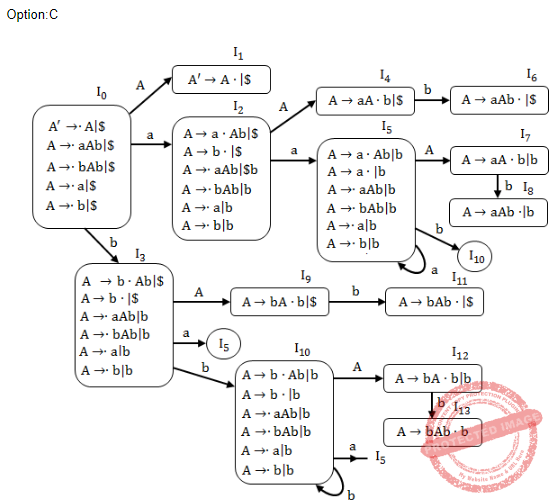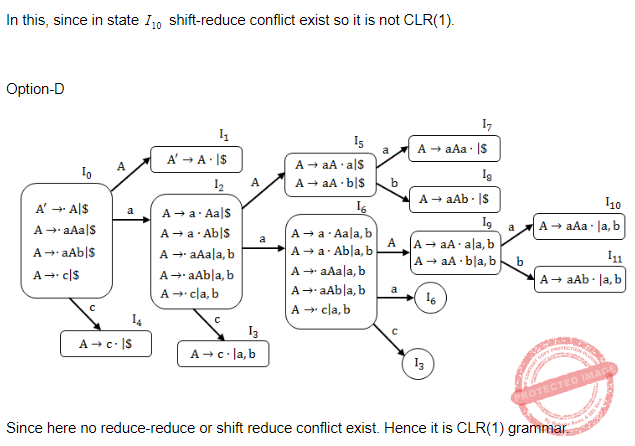Question 84
At the end of parsing,
 A tokens are identified. B set of instructions are identified. C the syntactic groups are identified. D machine instructions are identified
Compiler-Design       Parsers       UGC NET CS 2008-june-Paper-2
Question 84 Explanation:
At end of parsing whether it is syntactically correct or not is identified So at end of parsing
1. tokens are identified
2. whether the given code is syntactically correct or not is identified.
 Question 85
A parse tree is an annotated parse tree if :
 A it shows attribute values at each node. B there are no inherited attributes. C it has synthesized nodes as terminal nodes. D every non-terminal nodes is an inherited attribute.
Compiler-Design       Parsers       UGC NET CS 2008-june-Paper-2
Question 85 Explanation:
A parse tree is an annotated parse tree if it shows attribute values at each node.
Features:
1. High level specification
2. Hides implementation details
3. Explicit order of evaluation is not specified
 Question 86
Top-down parsers are predictive parsers, because :
 A next tokens are predicted. B length of the parse tree is predicted beforehand C lowest node in the parse tree is predicted. D next lower level of the parse tree is predicted.
Compiler-Design       Parsers       UGC NET CS 2007-Dec-Paper-2
Question 86 Explanation:
→ Top-down parsers are predictive parsers, because next tokens are predicted.
→ Predictive parser is a recursive descent parser, which has the capability to predict which production is to be used to replace the input string.
→ The predictive parser does not suffer from backtracking.
→ Predictive parsing uses a stack and a parsing table to parse the input and generate a parse tree.
 Question 87
The parsing technique that avoids backtracking is :
 A Top - down parsing B Recursive - descent parsing C Predicative D Syntax tree
Compiler-Design       Parsers       UGC NET CS 2007 June-Paper-2
Question 87 Explanation:
→ Top-down parsers are predictive parsers, because next tokens are predicted.
→ Predictive parser is a recursive descent parser, which has the capability to predict which production is to be used to replace the input string.
→ The predictive parser does not suffer from backtracking.
→ Predictive parsing uses a stack and a parsing table to parse the input and generate a parse tree.
 Question 88
A Top down Parser generates :
 A Rightmost derivation. B Rightmost derivation, in reverse. C Leftmost derivation. D Leftmost derivation in reverse.
Compiler-Design       Parsers       UGC NET CS 2007 June-Paper-2
Question 88 Explanation:
→ A Top down Parser generates leftmost derivation.
→ A bottom up parser generates rightmost derivation, in reverse.
 Question 89
Shift-reduce parser consists of
(a) input buffer
(b) stack
(c) parse table
choose the correct option from those given below:
 A (a) and (b) only B (a) and (c) only C (c) only D (a), (b) and (c)
Compiler-Design       Parsers       UGC NET June-2019 CS Paper-2
Question 89 Explanation:
Shift-reduce parser consists of
(a) input buffer
(b) stack
(c) parse table
 Question 90

Which of the following is/are FALSE?

I) Operator precedence parser works on ambiguous grammar

II) Top-down parser works on left recursive, unambiguous and deterministic grammar

III) LL(I) is a non-recursive descent parser

IV) CLR(I) is the most powerful parser
 A Only II B I, II, III and IV C ll and IV D I, III and IV
Compiler-Design       Parsers       CIL Part - B
Question 90 Explanation:
I) TRUE: Operator precedence parser works on ambiguous grammar
II) FALSE: Top-down parser works on left recursive, unambiguous and deterministic grammar
Ill) TRUE: LL(I) is a non-recursive descent parser
IV) TRUE: CLR(I) is the most powerful parser
 Question 91
What will be the "First" and "Follow" of E and F for the following grammar?
E->TE’
E’->+TE’/ε
T->FT’
T’->*FT’/ε
F->id/(E)
 A First(E)={id, (,ε},follow(E)={ε,) }, First(F)={id,),\$}, Follow(F)={*,\$,(} B First(E)={id, ( },follow(E)={\$,) }, First(F)={id,(}, Follow(F)={*,\$,),+} C First(E)={id, (,ε},follow(E)={ε,) }, First(F)={id,)}, Follow(F)={*,\$,(,+ } D First(E)={id, )},follow(E)={\$,) }, First(F)={id,(,\$}, Follow(F)={*,\$,),+}
Compiler-Design       Parsers       CIL Part - B
Question 91 Explanation:

First (∊) = { id, ( }

Follow (∊) = { \$, ) }

First (F) = { id, ( }

Follow (F) = { *, +, \$, ) }
 Question 92

Which of the following statements is TRUE for the grammar given below?

S->(L)/a

L->L.S/S
 A The grammar can be parsed by LR(0) parser only B The grammar can be parsed by LR(0) and SLR(1) parsers C The grammar can be parsed by LL(1) parser only D The grammar can be parsed by LL(1) and LR(0) parsers
Compiler-Design       Parsers       CIL Part - B
Question 92 Explanation:→ The given grammar can be parsed by LR(0) grammar because there is no S-R conflict or R-R conflict. → Since the grammar can be parsed by LR(0) parser hence we can say SLR(1) parser can also parse it.
 Question 93

The number of tokens in the following

“C” language statement is:

printf(“The number of tokens are %d”, &tcount);
 A 8 B 9 C 10 D 11
Compiler-Design       Parsers       CIL Part - B
Question 93 Explanation:Question 94
Given the grammar
s→ T*S | T
T → U + T | U
U → a | b
Which of the following statements is wrong?
 A Grammar is not ambiguous B Priority of + over * is ensured C Right to left evaluation of * and + happens D None of these
Compiler-Design       Parsers       ISRO CS 2020       Video-Explanation
Question 94 Explanation:
The grammar is unambiguous as it can be parsed by CLR(1) parser.
S-> T*S , since S has right recursion so * is right associative
T-> U+T , since T has right recursion so + is right associative
Hence right to left evaluation of * and + will happen.
+ is having more precedence than * so the precedence of + and * is clearly defined.
 Question 95
Consider the grammar with non-terminals N = {S,C,S1}, terminals T = {a,b,i,t,e}, with S as the start symbol, and the following set of rules: S -- > iCtSS1|a S1 -- > eS | • C -- > b The grammar is NOT LL(1) because:
 A It is left recursive B It is right recursive C It is ambiguous D It is not context-free
Compiler-Design       Parsers       APPSC-2016-DL-CS
Question 95 Explanation: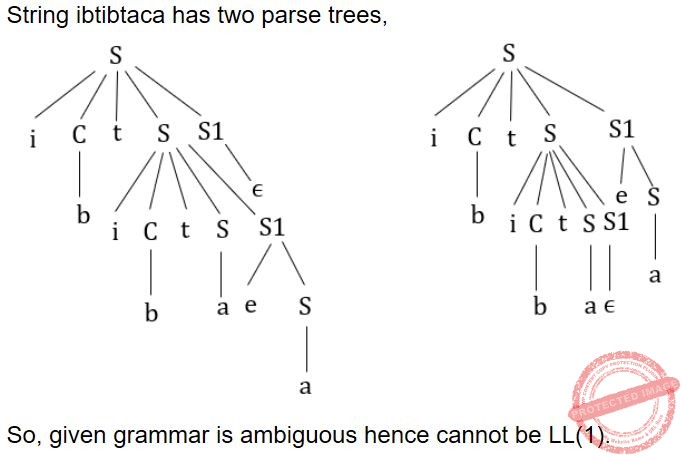Question 96
For the grammar below, a partial LL(1) parsing table is also presented along with the grammar. Entries that need to be filled are indicated as E1, E2, and E3. If the empty strinp, \$indicates end of input, and, | separates alternate right hand sides of productions S->aAbB|bAaB|ε A->S B->S
 a b \$ S E1 E2 S->ε A A->S A->S error B B->S B->S E3

 A FIST(A)=(a,b,\$) FIRST(A)={A,B} FIRST(B) = {a,b,ε} FOLLOW(A)={A,B} FOLLOW(A) = {A,b} FOLLOW(B)={A,b,\$) FOLLOW(B) = {\$} FIRST(B) = {a,b,ε} FOLLOW(A) = {A,b} B FOLLOW(B) = {\$} C FIRST(A) = {a,b,ε} = FIRST(B) D FIRST(A) = {A,B} = FIRST(B) FOLLOW(A)={a,b} FOLLOW(A)={a,b}
Compiler-Design       Parsers       APPSC-2016-DL-CS
 Question 97
Which one of the following is a top-down parser?
 A Recursive descent parser. B Operator precedence parser. C An LR(k) parser. D An LALR(k) parser
Compiler-Design       Parsers       APPSC-2016-DL-CS
Question 97 Explanation:
Out of the given options only recursive decent parser is the top down parser.
 Question 98
Which one of the following is True at any valid state in shift-reduce parsing?
 A Viable preflxes appear only at the bottom of the stack and not inside B Viable prefixes appear only at the top of the stack and not inside C The stack contains only a set of viable prefixes D The stack never contains viable prefixes
Compiler-Design       Parsers       APPSC-2016-DL-CS
Question 98 Explanation:
In SR parsing the stack contains only viable prefixes.
 Question 99

Consider the following grammar

` S → m | mn | mno `
Choose correct statement from the following:

 A The grammar is LL(4) B The grammar is LL(3) C The grammar is LL(2) D The grammar is LL(1)
Compiler-Design       Parsers       CIL 2020
Question 99 Explanation:
Intersection of first() of any two production of S is ‘m’ which is not equal to {empty}, so not LL(1).
Intersection of second() of second and third production of S is ‘n’ which is not equal to {empty}, so not LL(2).
Intersection of third() of any two production of S is equal to {empty}, so yes LL(3).
 Question 100

Which of the following derivations does a top-down parser use while parsing a input string?
The input is assumed to be scanned in left to right order.

 A Topmost derivation B Leftmost derivation traced out in reverse C Leftmost derivation D Rightmost derivation
Compiler-Design       Parsers       CIL 2020
Question 100 Explanation:
Top down parser use left most derivation while Bottom up parser use reverse of right most derivation.
 Question 101

Which of the following statement is true?

 A The parsers canonical LR and LALR have the same power B LALR parser is more important than canonical LR parser C SLR parser is more powerful than LALR parser D Canonical LR parser is more powerful than LALR parser
Compiler-Design       Parsers       CIL 2020
Question 101 Explanation:
The power of parsers is given by
CLR > LALR > SLR
 Question 102

Which grammar rules violate the requirement of the operator grammar? A,B,C are variables and a,b,c are terminals.

```I. A → BC
II. A → CcBb
III. A → BaC
IV. A → ε ```
 A I only B I and II C I and III D I and IV
Compiler-Design       Parsers       CIL 2020
Question 102 Explanation:
In operator grammar there should be no epsilon or empty string in the production, and also there should be no two non-terminals adjacent to each other in the LHS.
(I) violates the operator grammar condition as it contains two adjacent non terminals on LHS.
(IV) violates the operator grammar condition as it contains epsilon.
 Question 103

Write true (T) / false (F) for each of the following statements:
A. Symbol table is used only in the first phase of a compiler.
B. A pretty printer analyses a program and prints it in such a way that the structure of the program becomes early visible.
C. YACC build up LALR parsing table
D. If a grammar is LALR(1), it is not necessarily SLR(1)

 A T T F T B F T T T C T T T F D F T T F
Compiler-Design       Parsers       CIL 2020
Question 103 Explanation:
A- False, Symbol table can be used in all the phases of the compiler.
B- True.
C- True, YACC is a tool to build the LALR parsing table.
D- True. If a grammar is LALR(1), it is not necessarily SLR(1). Below diagram is the parsers according to their powers and it justifies the given statement.
 Question 104

The following grammar is:

```S →  Aa | bAc | Bc | bBa
A →  d
B →  d ```
 A Not LR(1) but LALR(1) B Neither LR(1) nor LALR(1) C LR(1) and LALR(1) D LR(1) but not LALR(1)
Compiler-Design       Parsers       CIL 2020
Question 104 Explanation:
Let's draw automata for LR(1),Since there is no RR or SR conflict hence the given grammar is LR(1).
Now, for LALR(1) let’s combine I1 & I3,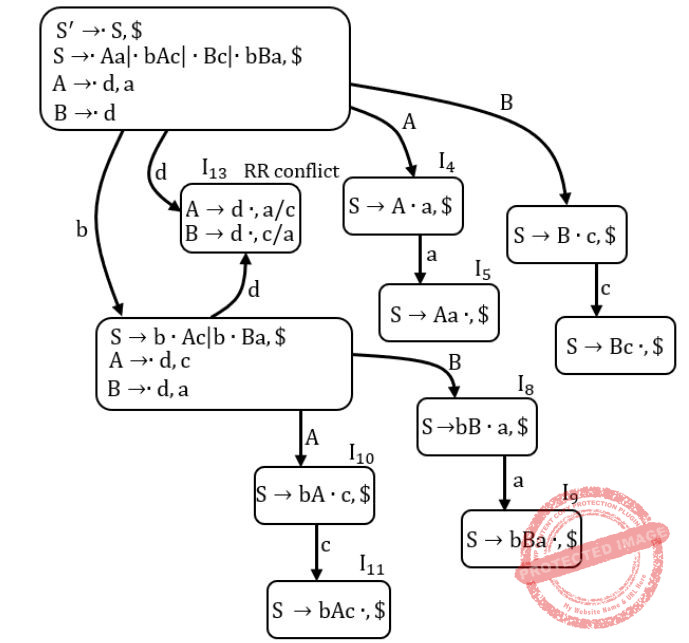Since there is RR conflict in state I13, hence not LALR(1).
 Question 105

Consider the grammar

```S --> AbBaCc |ε
A --> aAb | ba
B --> bBC | cb
C --> cCa | ac ```
Find the first() of S

 A {a, b, ε} B {ε} C {a,ε} D {a,b,c,ε}
Compiler-Design       Parsers       CIL 2020
Question 105 Explanation:
First(S) = First(A) ∪ {∊}
First(A) = {a,b}
∴ First(S) = {a,b} ∪ {∊}
= {a,b,∊}
 Question 106

The following grammar is:

```S →  Aa | b Ac | dc | bda
A →  a ```
 A Neither LALR(1) nor SLR(1) B LALR(1) but not SLR(1) C Not LALR(1) but SLR(1) D LALR(1) and SLR(1)
Compiler-Design       Parsers       CIL 2020
Question 106 Explanation:
Let’s check for SLR(1),No SR or RR conflict, hence SLR(1).
Every SLR(1) grammar is LALR(1).
Hence given grammar is also LALR(1).
 Question 107
Which of the following statements is false?
 A An unambiguous grammar has the same leftmost and rightmost derivation B An LL(1) parser is a top-down parser C LALR is more powerful than SLR D An unambiguous grammar can never be LR(k) for any k
Compiler-Design       Parsers       APPSC-2012-DL-CS
Question 107 Explanation:
1 is false because unambiguous grammar need not have the same leftmost and rightmost derivation.
4 is false because the correct statement is an ambiguous grammar can never be LR(K) for any k.
 Question 108
Which of the following derivations does a top-down parser use while parsing an input string? The input is assumed to be scanned in left to right order.
 A Leftmost Derivation B Leftmost Derivation traced out in reverse C Rightmost Derivation D Rightmost Derivation traced out in reverse
Compiler-Design       Parsers       APPSC-2012-DL-CS
Question 108 Explanation:
Top down parser uses leftmost derivation while parsing an input, while bottom-up parser uses reverse of rightmost derivation while parsing an input.
 Question 109
The grammar A→AA|(A)|∈ is not suitable for predictive parsing because the grammar is:
 A Ambiguous B Left - Recursive C Right Recursive D An operator grammar
Compiler-Design       Parsers       TNPSC-2017-Polytechnic-CS
Question 109 Explanation:
Let’s draw parse tree for string ‘( )’Since for the given string two parse trees are possible. So the given grammar is ambiguous.
 Question 110
Consider the grammar
S→AS/b A→SA / a then Closure (S'→.S,\$) is:
 A S1→.S,\$ S→.AS,\$ / a/b S→.b,\$/a/b A→.SA, a/b A→.a,a /b B S1→.S,\$ S→.AS,\$ s/ b S→.b,\$ / b C S1→.S,\$ S→.AS,\$ / a/b S→.AS,\$ / a/b S→.b,\$ / a/b D S1→.S,\$ S→.AS,\$ S→.b,\$
Compiler-Design       Parsers       TNPSC-2017-Polytechnic-CS
Question 110 Explanation:
The given grammar is
S → AS|b
A → SA|a
Now closure for S →.S, \$,
S’ → .S, \$
S → .AS|.b, \$
A → .SA|.a, a|b
S → .AS|.b, a|b

S → .S, \$
S → .AS|.b, \$|a|b
A → .SA|.a, a|b
 Question 111
Consider the parse tree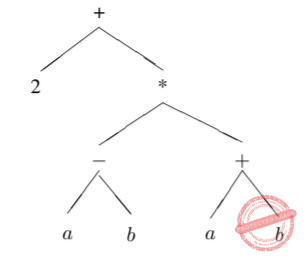Assume that * has higher precedence than +, − and operators associate right to left (i.e. a + b + c =(a + (b + c))). Consider
(i) 2 + a − b
(ii) 2 + a − b ∗ a + b
(iii) (2 + ((a − b) ∗ (a + b)))
(iv) 2 + (a − b) ∗ (a + b)
The parse tree corresponds to
 A Expression (i) B Expression (ii) C Expression (iv) only D Expression (ii), (iii) and (iv) E Expressions (iii) and (iv) only
Compiler-Design       Parsers       TIFR PHD CS & SS 2012
 Question 112
Which of the following correctly describes LR(k) parsing?
 A The input string is alternately scanned left to right and right to left with k reversals. B Input string is scanned once Left to Right with rightmost derivation and k symbol look-ahead C LR(k) grammers are expressively as powerful as context-free grammers. D Parser makes k left-to-right passes over input string E Input string is scanned from left to right once with k symbol to the right as look-ahead to give left-most derivation.
Compiler-Design       Parsers       TIFR PHD CS & SS 2012
 Question 113
Shift reduce parsing can also be called as :
 A Reverse of the Right Most Derivation B Right Most Derivation C Left Most Derivation D None of the options
Compiler-Design       Parsers       NIC-NIELIT STA 2020
Question 113 Explanation:
Shift reduce parsing can also be called as Reverse of the Right Most Derivation.
It is a bottom up parsing technique.
 Question 114
The LL(1) and LR(0) techniques are ______.
 A Both same in power B Both simulate reverse of rightmost derivation C Both simulate reverse of left most derivation D Incomparable
Compiler-Design       Parsers       NIC-NIELIT STA 2020
Question 114 Explanation:
LL(1) is a Top down parser that parsers the leftmost derivation.
LL(0) is a Bottom up parser that parses the reverse of rightmost derivation.
 Question 115
Type of conflicts that can arise in LR(0) techniques are ______.
 A Shift-reduce conflict B Shift-Shift conflict C Both Shift-reduce conflict & Shift-Shift conflict D None of the options
Compiler-Design       Parsers       NIC-NIELIT STA 2020
Question 115 Explanation:
Type of conflicts that can arise in LR(0) techniques are Shift-reduce or Reduce-reduce conflicts:
 Question 116
Assume that the SLR parser for a grammar G has n1 states and the LALR parser for G has n2 states. The relationship between n1 and n2 is:
 A n1 is necessarily less than n2 B n1 is necessarily equal to n2 C n1 is necessarily greater than n2 D none of the options
Compiler-Design       Parsers       NIC-NIELIT STA 2020
Question 116 Explanation:
No. of states in SLR and LALR are equal and no. of states in SLR and LALR are less than or equal to LR(1).
There are 116 questions to complete.